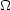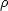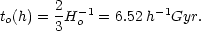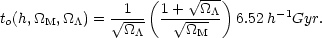7.8. Observational Constraints from the CMB

Our general relativistic description of the Universe can be divided into two parts, those parameters likei and H which describe the global properties of the model and those parameters like ns and A which describe the perturbations to the global properties and hence describe the large scale structure (Table 1).

In the context of general relativity and the hot big bang model, cosmological parameters are the numbers that, when inserted into the Friedmann equation, best describe our particular observable universe. These include Hubble's constant H (or h = H / 100 km s-1 Mpc -1), the cosmological constant=/ 3H2, geometryk = - k / H2 R2, the density of matter,M =CDM +baryon =CDM /c +baryon /c and the density of relativistic matterrel =+. Estimates for these have been derived from hundreds of observations and analyses. Various methods to extract cosmological parameters from cosmic microwave background (CMB) and non-CMB observations are forming an ever-tightening network of interlocking constraints. CMB observations now tightly constraink, while type Ia supernovae observations tightly constrain the deceleration parameter qo. Since lines of constantk and constant qo are nearly orthogonal in theM -plane, combining these measurements optimally constrains our Universe to a small region of parameter space.

The upper limit on the energy density of neutrinos comes from the shape of the small scale power spectrum. If neutrinos make a significant contribution to the density, they suppress the growth of small scale structure by free-streaming out of over-densities. The CMB power spectrum is not sensitive to such small scale power or its suppression, and is not a good way to constrain. And yet the best limits oncome from the WMAP normalization of the CMB power spectrum used to normalize the power spectrum of galaxies from the 2dF redshift survey (Bennett et al. 2003).

The parameters in Table 1 are not independent of each other. For example, the age of the Universe, to = h-1 f (M,). Ifm = 1 as had been assumed by most theorists until about 1998, then the age of the Universe would be simple:(36)

However, current best estimates of the matter and vacuum energy densities are (M,) = (0.27, 0.73). For such flat universes (=M += 1) we have (Carroll et al. 1992):(37)

for to(h = 0.71,M = 0.27,= 0.73) = 13.7 Gyr.

If the Universe is to make sense, independent determinations of,M and h and the minimum age of the Universe must be consistent with each other. This is now the case (Lineweaver 1999). Presumably we live in a universe which corresponds to a single point in multidimensional parameter space. Estimates of h from HST Cepheids and the CMB overlap. Deuterium and CMB determinations ofbaryon h2 are consistent. Regions of theM -plane favored by supernovae and CMB overlap with each other and with other independent constraints (e.g. Lineweaver 1998). The geometry of the Universe does not seem to be like the surface of a ball (k < 0) nor like a saddle (k > 0) but seems to be flat (k0) to the precision of our current observations.

There has been some speculation recently that the evidence foris really evidence for some form of stranger dark energy (dubbed `quintessence') that we have been incorrectly interpreting as. The evidence so far indicates that the cosmological constant interpretation fits the data as well as or better than an explanation based on quintessence.

 Composition of Universea Total densityo 1.02 ± 0.02 Vacuum energy density0.73 ± 0.04 Cold Dark Matter densityCDM 0.23 ± 0.04 Baryon densityb 0.044 ± 0.004 Neutrino density< 0.0147 95% CL Photon density4.8 ± 0.014 × 10-5 Fluctuations Spectrum normalizationb A 0.833+0.086-0.083 Scalar spectral indexb ns 0.93 ± 0.03 Running index slopeb dns / d lnk -0.031+0.016-0.018 Tensor-to-scalar ratioc r = T/S < 0.71 95% CL Evolution Hubble constant h 0.71+0.04-0.03 Age of Universe (Gyr) t0 13.7 ± 0.2 Redshift of matter-energy equality zeq 3233+194-210 Decoupling Redshift zdec 1089 ± 1 Decoupling epoch (kyr) tdec 379+8-7 Decoupling Surface Thickness (FWHM)zdec 195 ± 2 Decoupling duration (kyr)tdec 118+3-2 Reionization epoch (Myr, 95% CL)) tr 180+220-80 Reionization Redshift (95% CL) zr 20+10-9 Reionization optical depth0.17 ± 0.04 ai =i /c wherec = 3H2 / 8G b at a scale corresponding to wavenumber k0 = 0.05 Mpc-1 c at a scale corresponding to wavenumber k0 = 0.002 Mpc-1